# Derivation of Beer Lambert Law

Beer-Lambert Law derivation helps us to define the relationship of the intensity of visible UV radiation with the exact quantity of substance present. The derivation of Beer-Lambert Law has many applications in modern-day science. Used in modern-day labs for testing of medicines, organic chemistry and to test with quantification. These are some fields that this law finds its uses in.

## Beer-Lambert Law Statement

The Beer-Lambert law states that:

for a given material sample path length and concentration of the sample are directly proportional to the absorbance of the light.

The Beer-Lambert law is expressed as:

 A = εLc

where,

• A is the amount of light absorbed for a particular wavelength by the sample
• ε is the molar extinction coefficient
• L is the distance covered by the light through the solution
• c is the concentration of the absorbing species

Following is an equation to solve for molar extinction coefficient:

 $$\epsilon =\frac{A}{Lc}$$

But Beer-Lambert law is a combination of two different laws: Beer’s law and Lambert law.

### What is Beer’s Law?

Beer’s law was stated by August Beer which states that concentration and absorbance are directly proportional to each other.

### What is Lambert Law?

Johann Heinrich Lambert stated Lambert law. It states that absorbance and path length are directly proportional.

## Beer-Lambert Law Formula

 $$I=I_{0}e^{-\mu (x)}$$

Where,

• I is the intensity
• I0 is the initial intensity
• x is the depth in meters
• 𝜇 is the coefficient of absorption

Following is the table explaining concepts related to Physics laws

## Schematic Diagram of Beer-Lambert LawAbsorption of energy causes the absorption of light as well usually by electrons. Different forms of light such as visible light and ultraviolet light get absorbed in this process. Therefore, change in the intensity of light due to absorption, interference, and scattering leads to:

ΔI = I0IT

The following equations are necessary for us to obtain our ultimate derivative equation. Transmittance is measured as the ratio of light passing through a substance. It can be calculated as IT/I0. To calculate the of transmittance percentage we can do so by:Percent Transmittance Another key metric is absorbance that is defined as the amount of light absorbed. This is usually calculated as the negative of transmittance and is given by: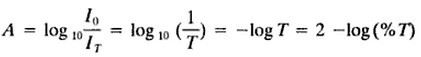Absorbance (A) The rate of decrease in the intensity of light with the thickness of the material the light is directly proportional to the intensity of the incident light. Mathematically, it can be expressed as: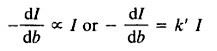As k’= Proportionality constant
Taking in the reciprocal of the equation we get,Integrating the above equation we also get,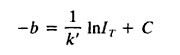In the above equation, b and C is the constant of integration and IT is the intensity being transmitted at the thickness

In order to solve the above equation with the constant of integration, we then get,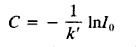While solving for C in the equation will give us,Converting to log10 we get,Rearranging the equation we get,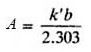Thus, Lambert’s law was formed and it states that the monochromatic radiation changes exponentially and decreases when it passes through a medium of uniform thickness.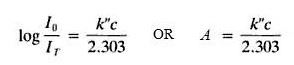Thus, this concludes the derivation of Beer-Lambert law. This goes to show you that in order to derive a particular law, there are a lot of different equations that need to be found out first, to achieve the ultimate result.

### Examples of Beer-Lambert Law

Q1. Determine the relative amount of light that is absorbed by the sample if the absorbance of the sample is 1 at a specific wavelength.

According to the definition of absorbance;

A = log10 (I0/I)

The equation is rearranged to determine the relative loss of intensity

10A = I0/I

10-A= I/I0

Substituting the value of A = 1

1-I/I0 = 1 – 10-1 = 1 – 1/10 = 0.9

Therefore, we can say that 90% of the light is absorbed and 10% of light is transmitted.

Q2. Determine the concentration of glycogen-iodine complex if the transmission of light is 40%. Also, the absorption coefficient is 0.20 at 450 nm. The size of the cuvette is 2 cm.

Using Beer-Lambert’s law, we know that

-log(It) = -log10(0.4) = (0.20)(c)(2)

c = 0.994

## Frequently Asked Questions – FAQs

### Why absorbance has no unit?

Absorbance has no unit because when the light passes through a material, none of the light is absorbed by the material, therefore, the absorbance of the material is zero. Since there is no absorbance of the light, the transmission from the material is 100%. However, the typical unit of absorbance is known as “absorbance units” which is abbreviated as AU and has no dimension.

### What are the limitations of Beer-Lambert law?

Following are the limitations of Beer-Lambert law:

• A diluted solution is used
• There shouldn’t be a scattering of the light beam
• Monochromatic electromagnetic radiation should be used

### Why does Beer-Lambert law fails at higher concentrations?

Beer-Lambert law fails at higher concentrations because the linearity of the law is limited to chemical and instrumental factors. When the solution has higher concentrations, the proximity between the molecules of the solution is so close that there are deviations in the absorptivity. Also, when the concentration is high, the refractive index changes.

### What is Beer-Lambert’s law for absorption spectroscopy?

Beer-Lambert’s law for absorption spectroscopy is a linear relationship between the absorbance and the concentration of an absorbing species. The states imply that type, as well as the concentration of the molecules, are necessary.

### State the situations when Beer’s law is not obeyed.

Following are the situations when Beer’s law is not obeyed:

• When different types of molecules are in equilibrium with each other.
• An association complex is formed by the solute and the solvent.
• When fluorescent compounds are used.
• When thermal equilibrium is attained between the excited state and the ground state.

Test your knowledge on Beer-Lambert law derivation